# Solving equations using interval bisection

Martin McBride, 2021-04-02
Tags solving equations interval bisection
Categories numerical methods pure mathematics

The previous article discussed using numerical methods to solve equations of the form $f(x) = 0$.

In this article we will look at one of the simplest methods, interval bisection.

## Example problem

As a simple example, we will solve the equation:

$$x^2 - 2 = 0$$

This equation has a solution $x = \sqrt 2$ (and an second solution $x = -\sqrt 2$). We will only look for the first solution. Although we already know the answer to the problem, it is still useful to work through the numerical solution to see how it works (and to gain an approximate value for the square root of 2).

To use the technique we need to have a rough idea of where the solution is. It is useful to sketch a graph of the function: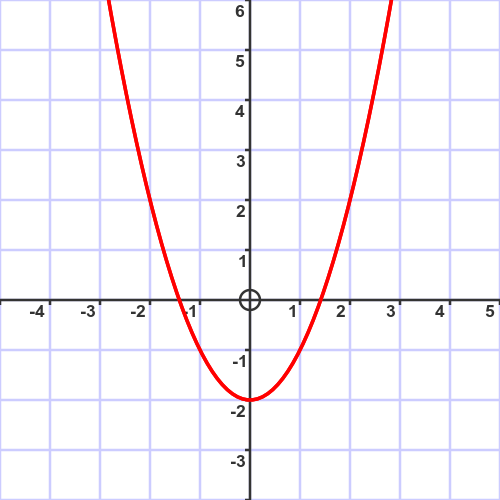We need to specify interval (ie a range of $x$ values) that contains exactly one solution. It doesn't have to be extremely accurate. For example, it is obvious from the graph that a solution exists between values $x=1$ and $x=2$. We write an interval as $[1, 2]$, meaning every number between 1 and 2.

The process of interval bisection proceeds as follows:

1. Start with an initial interval (1 to 2 in this case).
2. Divide the interval into two equal halves.
3. Determine whether the solution is in the lower half or the upper half.
4. Repeat step two with the selected half.

Steps 2 to 4 are repeated. On each pass, the interval width is halved, so the approximation becomes more accurate. When the result is accurate enough, the process ends.

## A graphical explanation of interval bisection

To gain an intuitive understanding of the process, we will go through a couple of iterations and show the results on a graph. This is for illustration only, you don't need to draw an accurate graph to use this method.

## First iteration

The first iteration starts with an interval $[1, 2]$. Here is the graph where we have zoomed in to the region of interest. a shows the line $x = 1$ and b shows the line $x = 2$: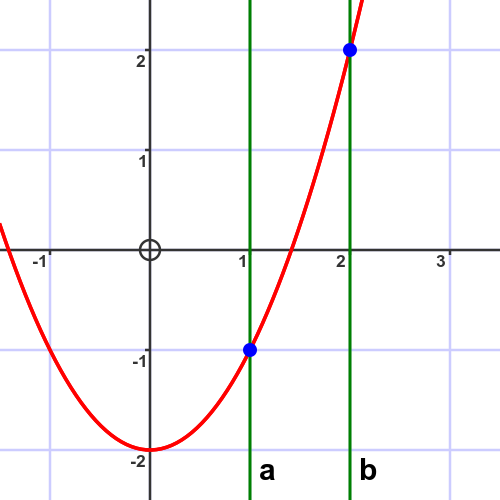We now find the midpoint of the interval:

$$m = (a + b) /2 = 1.5$$

We use this to divide the original interval in half to create 2 intervals $[a, m]$ and $[m, b]$: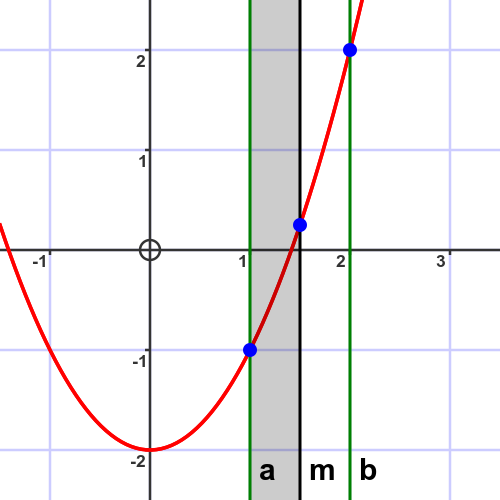The solution of the equation is the point where the curve crosses the x-axis. In this case, the solution is in the lower interval $[1, 1.5]$.

## Second iteration

The second iteration starts with the new interval $[1, 1.5]$. Again, we have zoomed in further: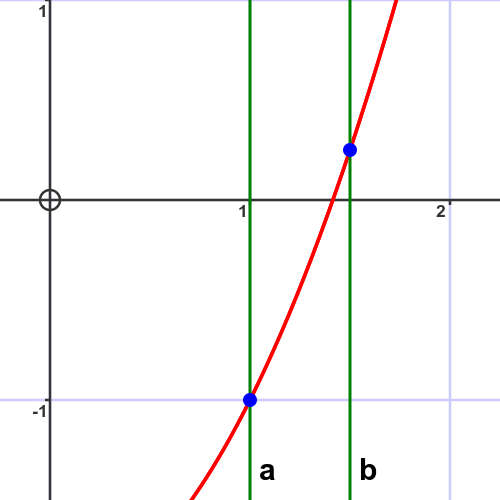The new midpoint is 1.25: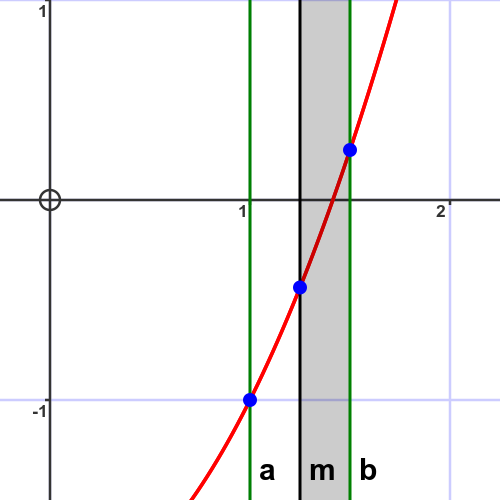This time the solution is in the upper interval $[1.25, 1.5]$.

## Third iteration

The third iteration starts with the new interval $[1.25, 1.5]$: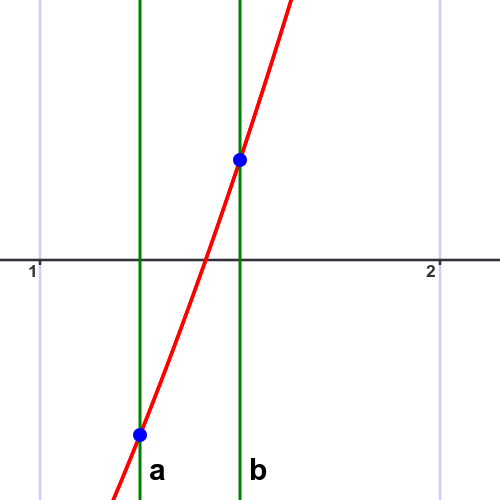The new midpoint is 1.375: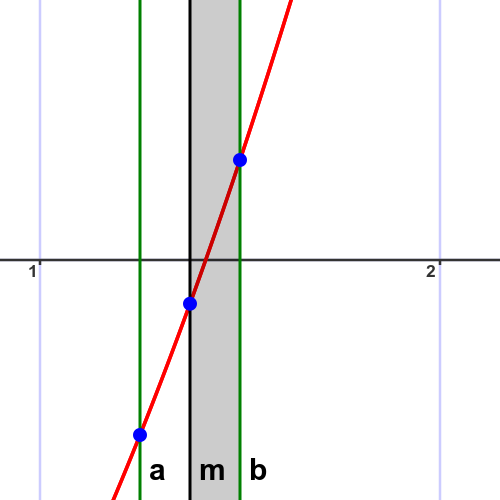The solution is in the upper interval $[1.375, 1.5]$.

On each iteration, the width of the interval is halved, starting at 1, then 0.5, then 0.25. After 10 iterations the interval width will be about 0.00097, so we will have calculated the square root of 2 to about 3 decimal places. After 20 iterations we will know the answer to around 6 decimal places, and after 30 we will be accurate to around 9 decimal places. Although this would be tedious (but not impossible) to do manually, for a computer it would take almost no time at all.

## Interval bisection by calculation

In the example above, we have relied on the fact that we have a very accurate graph of the function to determine whether the solution is in the first or second half of the interval. But is it possible to determine this by calculation alone. Of course, it is still useful to have an initial sketch graph to find a suitable starting interval.

For the first iteration, we know the values of $a$ and $b$. We can calculate $m$, and then the values of $f(a)$, $f(b)$, and $f(m)$. In this case, $f()$ is our function:

$$f(x) = x^2 - 2$$

Here is the calculation based on $a = 1$, $b = 2$

a b m f(a) f(b) f(m)
1 2 1.5 -1 2 0.25

Notice that $f(a)$ and $f(m)$ have opposite signs. This means that the root must be somewhere between $a$ and $m$.

For the second iteration, we therefore start with $a = 1$, $b = 1.5$ and calculate again:

a b m f(a) f(b) f(m)
1 1.5 1.25 -1 0.25 -0.4375

This time, $f(b)$ and $f(m)$ have opposite signs. This means that the root must be somewhere between $m$ and $b$.

For the third iteration, we therefore start with $a = 1.25$, $b = 1.5$, and so on.